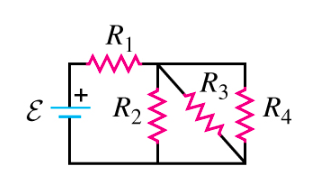# Problem: In the circuit of the figure (Figure 1), each resistor represents a light bulb. Let R1 = R2 = R3 = R4 = 4.43 Ω and let the EMF be 9.01 V. a. Find the power dissipated in the bulb R1.b. Find the power dissipated in the bulb R2.c. Find the power dissipated in the bulb R3.d. Find the power dissipated in the bulb R4.e. Which bulb or bulbs glows the brightest?

###### FREE Expert Solution

Power:

$\overline{){\mathbf{P}}{\mathbf{=}}{{\mathbf{i}}}^{{\mathbf{2}}}{\mathbf{R}}}$

The total current drawn from the battery is given by:

$\overline{){\mathbf{i}}{\mathbf{=}}\frac{\mathbf{V}}{{\mathbf{R}}_{\mathbf{e}\mathbf{q}}}}$

Resistors, R2, R3, and R4 are in parallel.

$\begin{array}{rcl}\frac{\mathbf{1}}{{\mathbf{R}}_{\mathbf{234}}}& \mathbf{=}& \frac{\mathbf{1}}{{\mathbf{R}}_{\mathbf{2}}}\mathbf{+}\frac{\mathbf{1}}{{\mathbf{R}}_{\mathbf{3}}}\mathbf{+}\frac{\mathbf{1}}{{\mathbf{R}}_{\mathbf{4}}}\\ \frac{\mathbf{1}}{{\mathbf{R}}_{\mathbf{234}}}& \mathbf{=}& \mathbf{=}\frac{\mathbf{1}}{\mathbf{4}\mathbf{.}\mathbf{43}}\mathbf{+}\frac{\mathbf{1}}{\mathbf{4}\mathbf{.}\mathbf{43}}\mathbf{+}\frac{\mathbf{1}}{\mathbf{4}\mathbf{.}\mathbf{43}}\\ \frac{\mathbf{1}}{{\mathbf{R}}_{\mathbf{234}}}& \mathbf{=}& \frac{\mathbf{300}}{\mathbf{443}}\\ {\mathbf{R}}_{\mathbf{234}}& \mathbf{=}& \frac{\mathbf{443}}{\mathbf{300}}\end{array}$

R234 = 443/300

83% (470 ratings)###### Problem Details

In the circuit of the figure (Figure 1), each resistor represents a light bulb. Let R1 = R2 = R3 = R4 = 4.43 Ω and let the EMF be 9.01 V.a. Find the power dissipated in the bulb R1.

b. Find the power dissipated in the bulb R2.

c. Find the power dissipated in the bulb R3.

d. Find the power dissipated in the bulb R4.

e. Which bulb or bulbs glows the brightest?

Frequently Asked Questions

What scientific concept do you need to know in order to solve this problem?

Our tutors have indicated that to solve this problem you will need to apply the Power in Circuits concept. You can view video lessons to learn Power in Circuits. Or if you need more Power in Circuits practice, you can also practice Power in Circuits practice problems.

What professor is this problem relevant for?

Based on our data, we think this problem is relevant for Professor Strauss' class at OU.